# The Moon Tilt & Terminator Illusions

Discussion in 'Flat Earth' started by Abishua, Nov 18, 2016.

1. ### Mick WestAdministratorStaff Member

It IS straight. The curved effect here is because you can't render a 180 degree scene with straight lines.

You should try this:

Sit on the floor about two feet from the middle of a wall (the longer the better), and look up at the corners of the room. See how the ceiling line goes UP from each corner when you look at that corner. Trace the line by pointing at it - you can see after a while how the line visually seems to go up and then curve down, just like these contrails do.

Go give it at go.

2. ### Mick WestAdministratorStaff Member

Dec 6, in the afternoon looks like a good time to duplicate that image in California: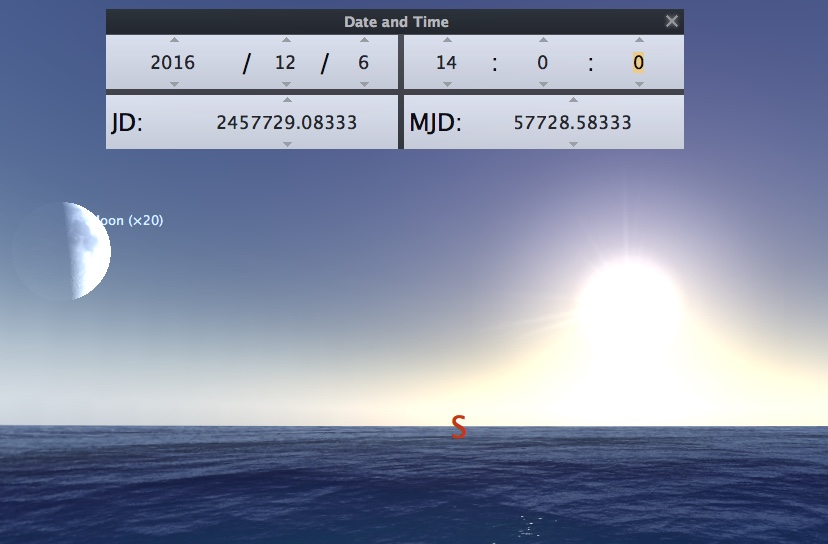3. ### Mick WestAdministratorStaff Member

Actually you could do it today or tomorrow, in the mid-morning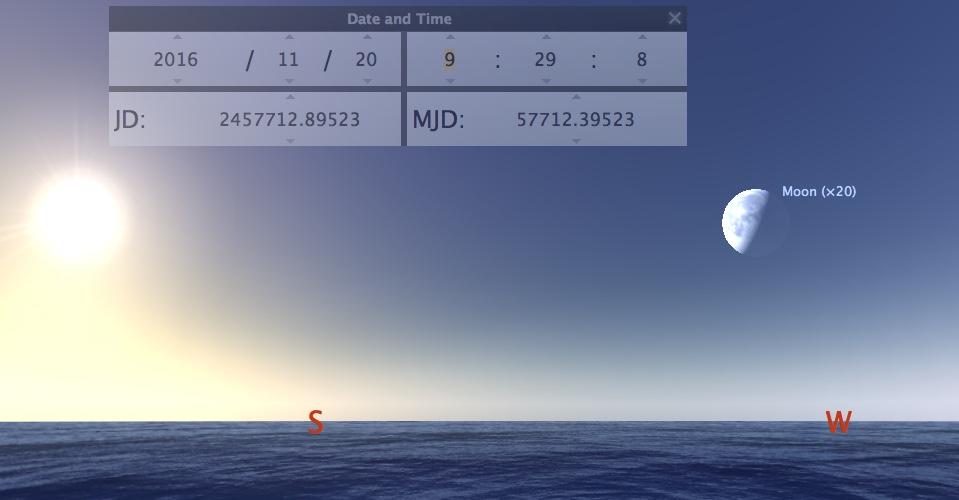4. ### Mick WestAdministratorStaff Member

Last edited: Nov 20, 2016
•Useful x 1
5. ### Mick WestAdministratorStaff Member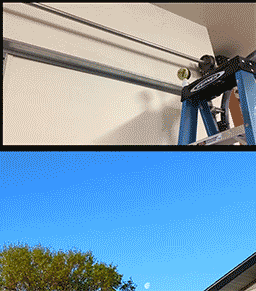•Like x 2
6. ### Z.W. WolfSenior Member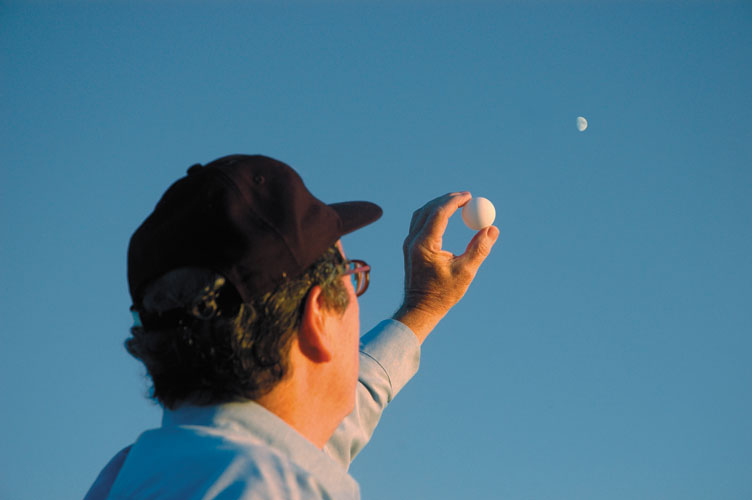I'd like to get a larger image of the moon.

The YT video in the OP was uploaded on 4/26/16. There was a waning gibbous moon (84% illuminated) that day. The sun and moon positions match a local time of 6:30 a.m. DST in Ann Arbor, MI on that day. We just passed that phase of the moon 2-3 days ago - although the moon phase was about 12 hours offset from that in April.

The next day the moon will be in that phase will be December 17, which happily is a Saturday. The moon phase will also be less offset and a good match to April. I'll try to get some photos in similar conditions on that day -sun and moon altitude about the same. Here in Las Vegas 7:40 a.m. looks pretty good. Any one want to join in?

(The author of that YT video has edited his video, and from what he says it's seemingly in response to this thread. You might want to take a look at the new version.)

Last edited: Nov 20, 2016
7. ### Z.W. WolfSenior Member

As Mick previously said, we've been mixing two issues here in this thread - and I was one of the prime culprits.

1. The Moon Tilt Illusion.

2. The Moon Phase Problem.

It's important to keep them separate and I apologize for not doing so. But photos with the sun-illuminated ball and the moon in the same frame will resolve both questions, especially if we catch the moon in different phases.

Last edited: Nov 20, 2016
8. ### Mick WestAdministratorStaff Member

My attempt: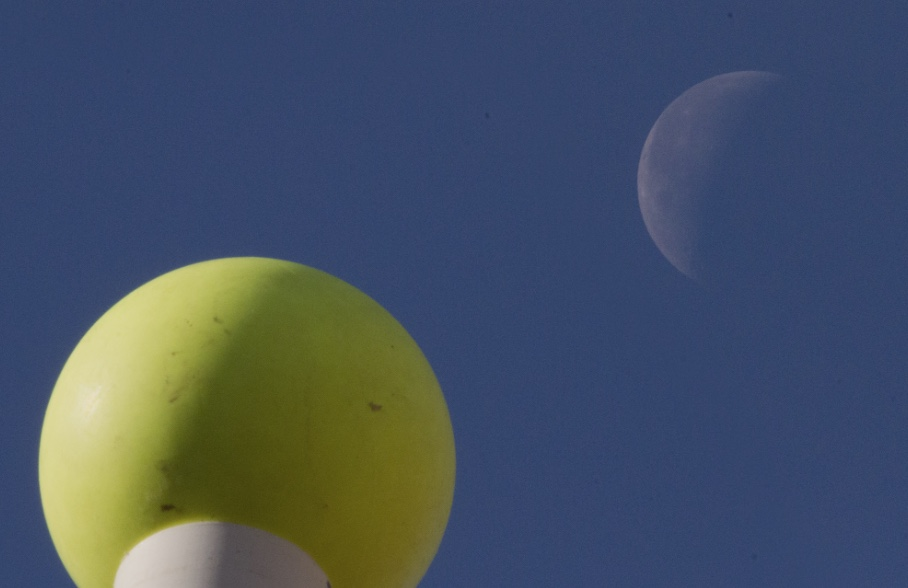Ball is on an 8 foot PVC tube. Right now the moon is so high that you can't get far enough away to get them both in focus with my 500mm. Hence the above is a composite image. A single shot focussed in-between looks like: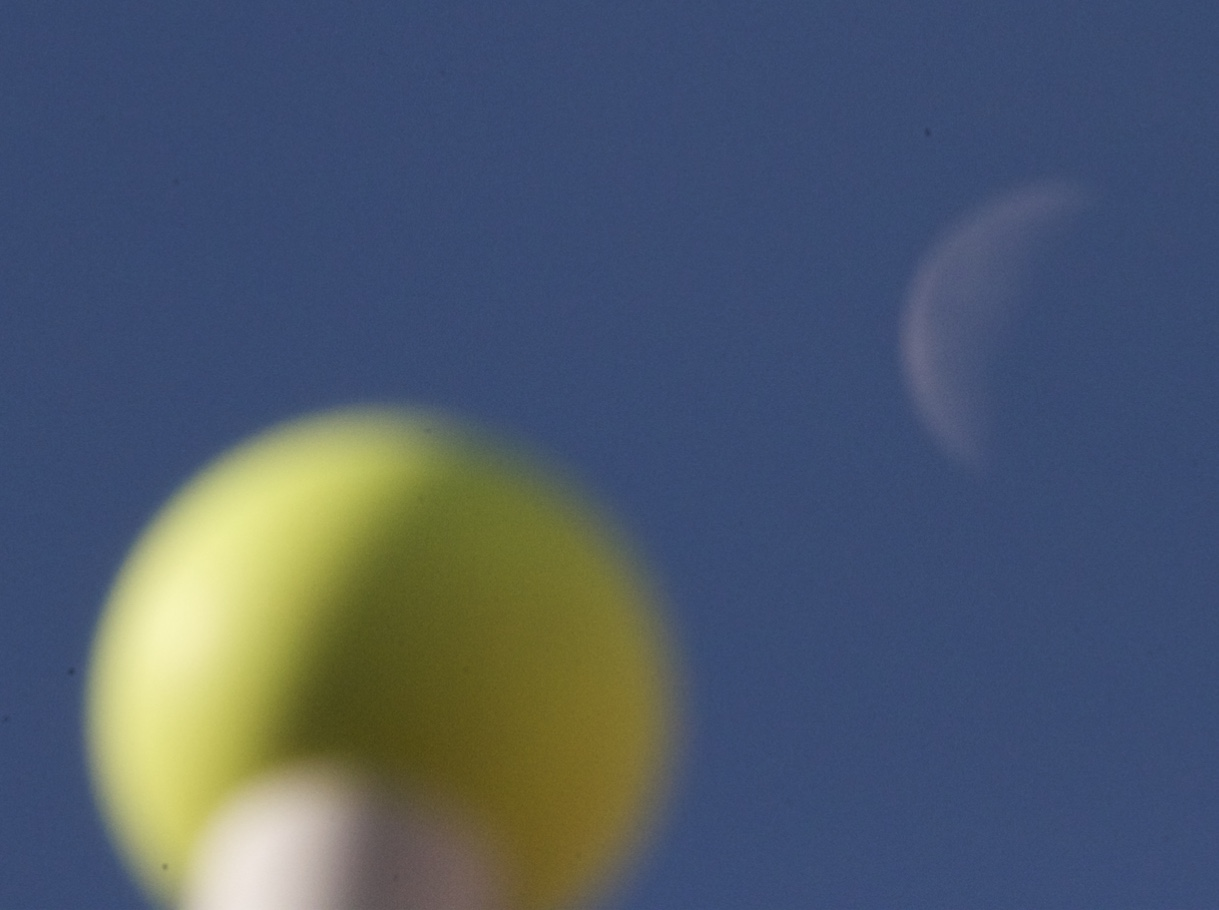Here's the layout, The terminator with the ball goes though the middle of the image, so it lines up with the sun.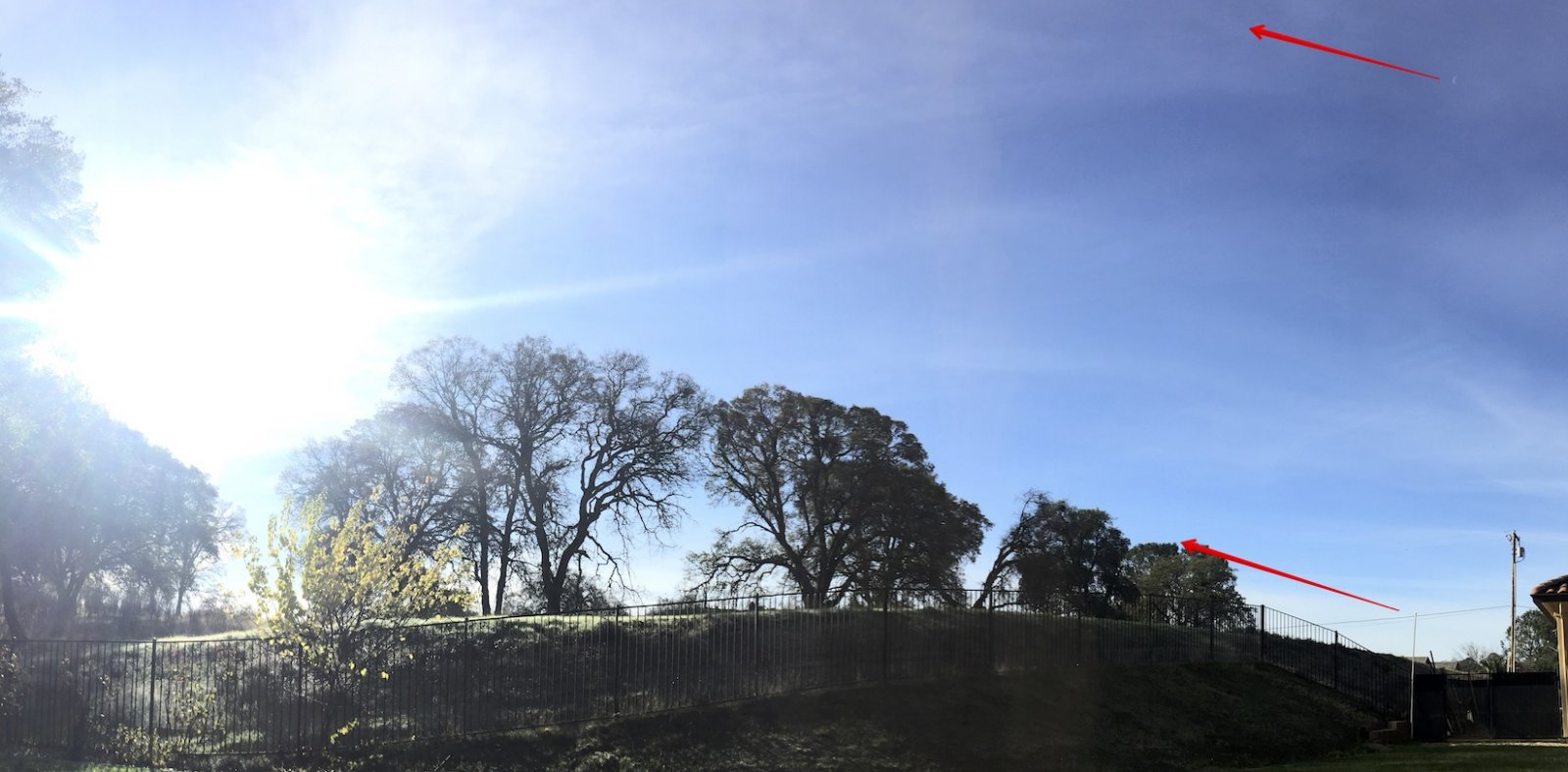Here's a better single image, 100mm at f/32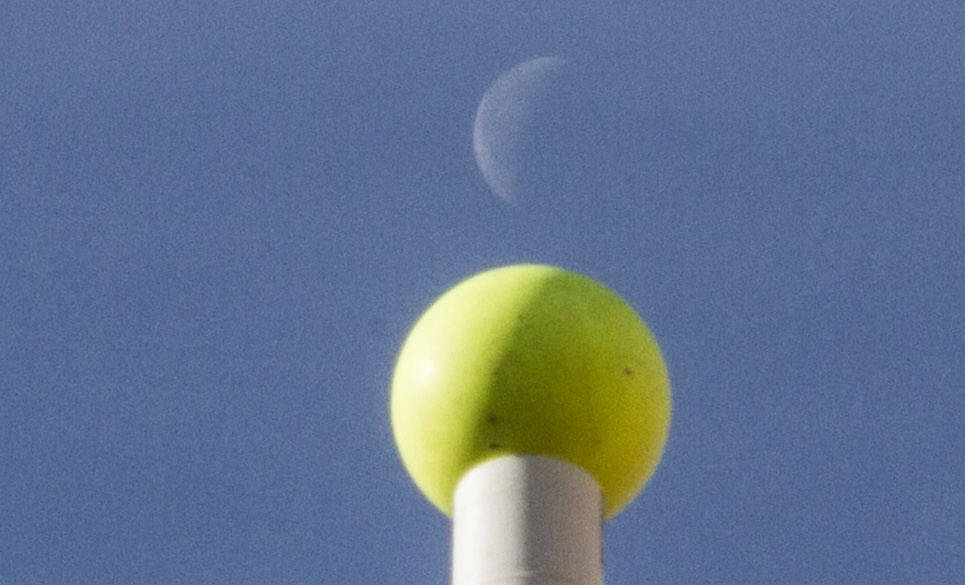Last edited: Nov 22, 2016
•Like x 2
9. ### Mick WestAdministratorStaff Member

A very slight difference between the two terminator lines is apparent. Probably due to a combinations of factors the sun light is not exactly parallel at the moon and the ball, and I'm closer to the ball, so there's some perspective distortion.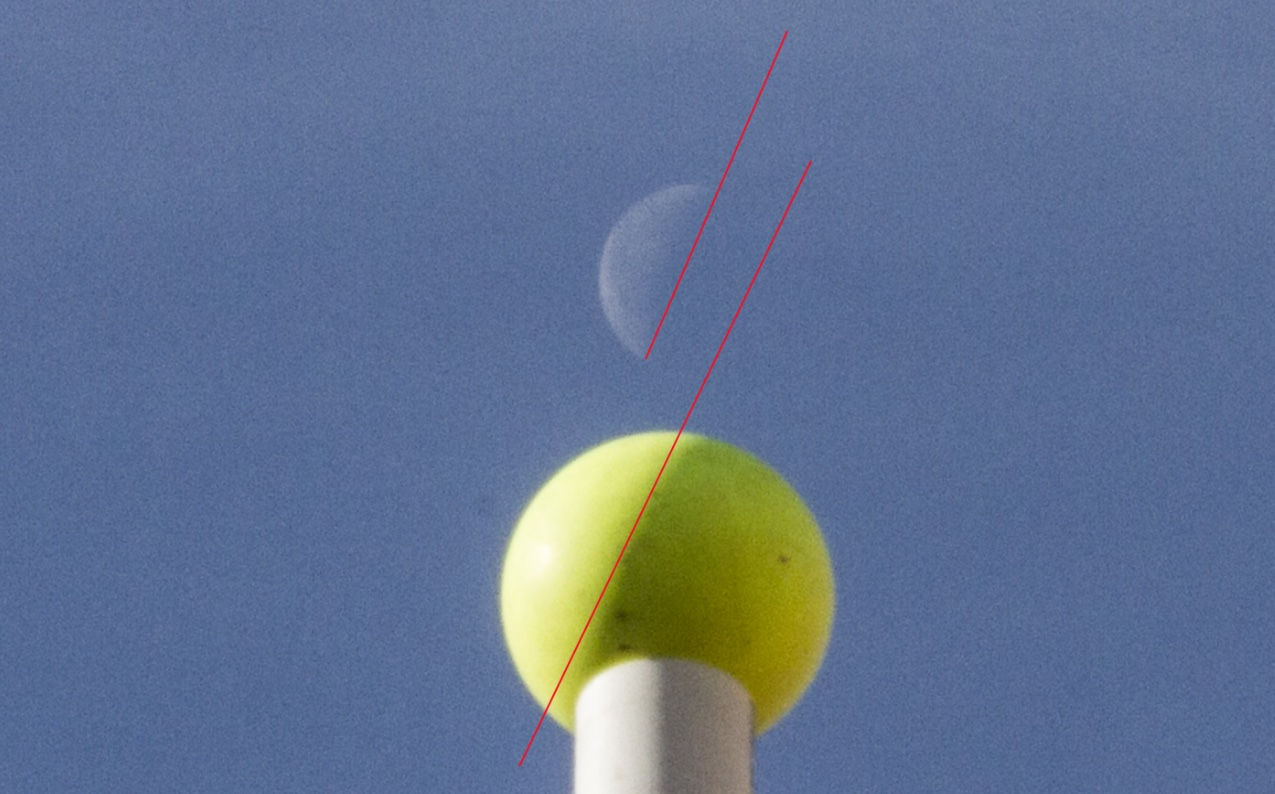•Like x 3
•Informative x 1
10. ### scientific601New Member

How would one go about calculating the angle of the terminator line? In the illustration above for example, I would say it is at roughly 64 degrees. It's driving me nuts - should I project sun's and moon's azimuth and elevation (from other sources) to derive 3D spacial coordinates and find an angle between them? There should be shortcut though. I can't seem to wrap my head around 3-D coordinate systems vs. appearance from the observer.

Please point me in the right direction... I don't mind the math/geometry formulas but I'm having trouble getting started. I have searched all over and no one seems to have solved this problem. This forum's topic has come closest to the problem so far. I've been trying to figure this out for years. If solved I would make a terminator angle webpage.

11. ### ApolloniusNew Member

The moon tilt illusion seems to be the same illusion that people are subject to when they assume that because Chicago and Beijing are at approximately the same latitude, the shortest route between them will follow a parallel of latitude. On the celestial sphere, the shortest path between two points is an arc of a great circle, so if the Moon is in the east and the Sun is in the west, the shortest line between them, i.e., the projection of a straight line between Sun and Moon on the sphere, will appear to go up closer to the zenith and then back down. The creator of the video in the OP mistakenly assumed that the straight-line path between Moon and Sun, both relatively low and separated by a wide angle, would be roughly parallel to the horizon rather than rising and then falling as a great-circle path would, and as the ecliptic and celestial Equator do.

Last edited: Jul 9, 2017
•Agree x 2
•Like x 1
12. ### Ross MarsdenSenior Member

I see that I "Liked" this comment in Nov 2016, but now I think that the red line drawn on the ball on the pole is not joining the points of the terminator exactly. One of the points (the lower one) is obscured by the pole, so we can't use that. The line should be tangential to the terminator line (curve) at it's mid point, not some place above that, as illustrated. Just saying.

•Like x 1
•Agree x 1
13. ### TrailblazerModeratorStaff Member

I took a simple hand-held picture using a ping pong ball and an iPhone when I was on holiday last month.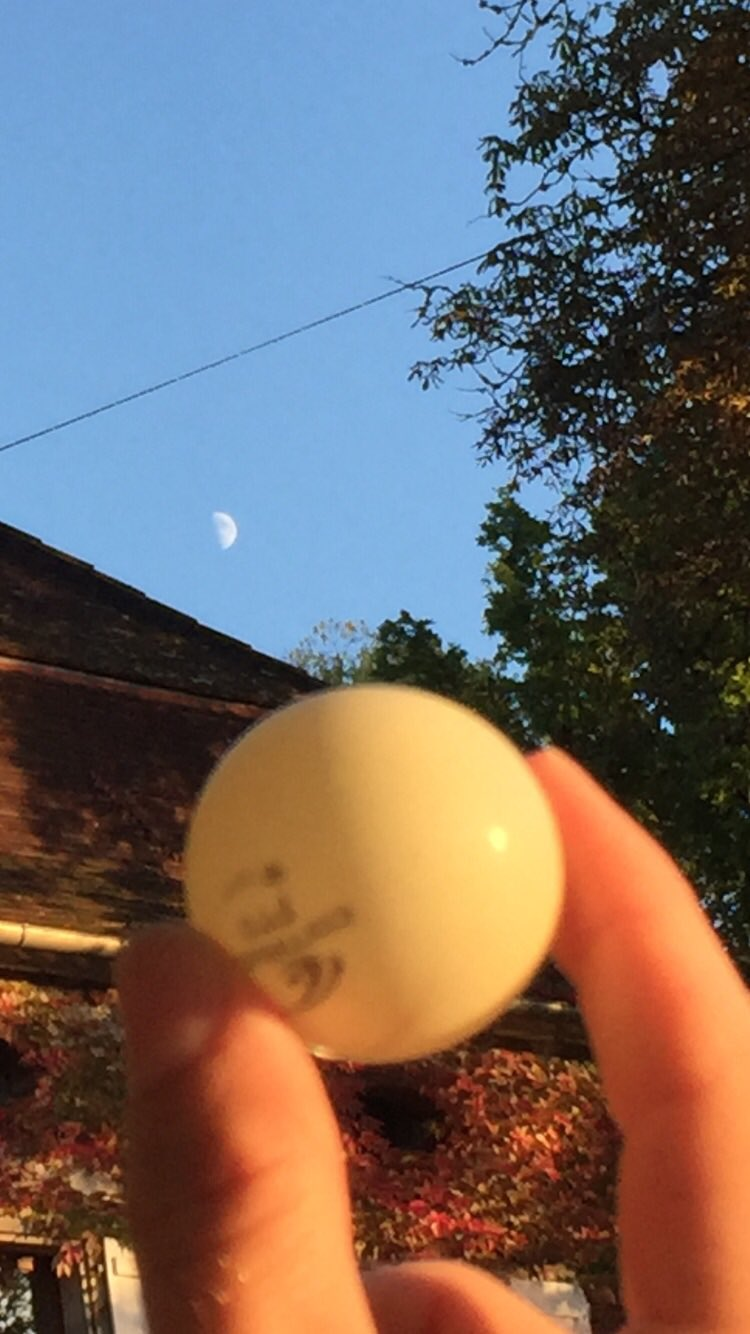•Like x 2
14. ### AstroActive Member

Here's the math. I incorporated it into this spreadsheet here:
http://dropcanvas.com/ecs9l
I presented it in a video here, but without going into too much detail about the math used:

Take the geocentric equatorial coordinates of the moon and add .25 degrees to declination (approximate radius of the moon). This will give you the equatorial coordinates of both the center point of the moon and the north point of the moon on the equatorial grid. Then calculate the position angle of the moon's bright limb relative to the north equatorial point. This is given by the following equation:

X = arctan((cos(declination of the sun)*Sin(right ascension of the sun - right ascension of the moon))/(cos(declination of the moon)*sin(declination of the sun)-sin(declination of the moon)*cos(declination of the sun)*cos(right ascension of the sun - right ascension of the moon)))

Then to calculate for field rotation, convert the geocentric equatorial coordinates of both the center point and the north point to horizon coordinates. This is given by the following formulae. For azimuth, the formula is:
tan(az)=(-sin(Hour angle in degrees +delta)*cos(declination))/(sin(declination)*cos(latitude)-cosnope(declination)*sin(latitude)*cos(hour angle in degrees+delta))
where delta is:
tan(delta)=(p*cos(theta')*sin(hour angle in degrees))/((distance of moon in km/6378.14)*cos(declination)-p*cos(theta')*cos(hour angle in degrees))
For altitude the formula is:
sin(altitude)=(sin(declination)*sin(latitude))/(cos(declination)*cos(latitude)*cos(hour angle in degrees))
Now once you have converted both the center point and north point to altitude and azimuth, take the difference of each (altitude and azimuth) between the north and center points to find delta alt and delta az. From there, calculate the angle of the line from the center point to north point relative to the horizon:
arctan(delta alt/delta az)
Then add the position angle of the moon (X). In order to express the resulting orientation angle of the moon relative to the horizon as I have in my spreadsheet (as an angle expressed as degrees from vertical from 0 to 90 degrees) follow these directions:
angle1 = arctan(delta alt/delta az)
If angle1 > 360, then take angle-360 = angle2, otherwise angle1 = angle2.
If angle2 >90 and less than or equal to 180, then take (90-angle2)+90 = angle3, otherwise angle2 = angle3.
If angle2 = angle3 and angle2 > 180 and less than or equal to 270, then take the absolute value of (180-angle2) = angle4, otherwise angle3 = angle4.
Finally, if angle4 = angle2 and angle 2 > 270, then take (90-(angle2-180)+90) = angle5, otherwise angle4 = angle5. Angle5 is the final answer and the apparent orientation of the moon relative to the horizon expressed as the angle from 0 to +90 degrees from vertical.

Hope this helps.

15. ### AstroActive Member

Meh, actually scratch that above, mostly. It's really quite a specific "use case" for the moon relatively near the horizon. Here's a much easier general approach you can use in a variety of calculators and spreadsheets (just be mindful of working in degrees, not radians). For the moon, just plug in topocentric coordinates as needed (take the center of the moon and add the apparent radius of about .25 degrees to get the north equatorial point, and subtract to get the south equatorial point). For the moon and other solar system bodies you'll still need to add this position angle formula to your result:
X = arctan((cos(declination of the sun)*Sin(right ascension of the sun - right ascension of the moon))/(cos(declination of the moon)*sin(declination of the sun)-sin(declination of the moon)*cos(declination of the sun)*cos(right ascension of the sun - right ascension of the moon)))

Here's a spreadsheet that does everything described below:
http://dropcanvas.com/x35q1
Now to calculate the field rotation for any imaginary line in the sky, the first step is to convert the right ascension and declination coordinates of both ends of the line to altitude and azimuth:
Altitude = asin(sin(Dec)*sin(latitude)+cos(Dec)*cos(latitude)*cos(Hour angle in degrees))
Where Dec = declination
latitude = observer's latitude
Hour angle in degrees = (Local Sidereal time in hours - (Right ascension in degrees /15))*15 degrees/hr

In my spreadsheet also included some correction factors for atmospheric refraction, but it's not really important unless you're dealing with areas of the sky very close to the horizon. See cells E25 and F25 of my spreadsheet for those equations.

A' = acos((sin(dec)-sin(latitude)*sin(altitude))/(cos(latitude)*cos(altitude)))
If sin(hour angle in degrees)<0, then altitude = A', otherwise altitude = 360-A'.

Once you have converted both sets of coordinates to local altitude and azimuth, use the following to calculate the angle of the line:
delta(a) = altitude 1 - altitude 2
delta(az) = (azimuth 1 - azimuth 2)*cos((altitude 1 + altitude 2)/2)

Then finally, the angle of the line relative to the horizon = atan2(x = delta(a), y = delta(az))